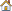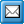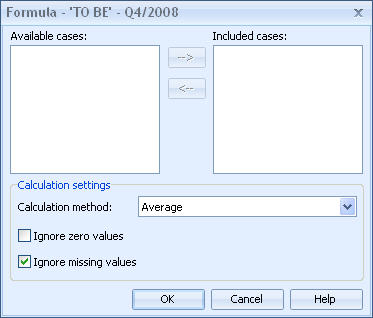﻿ Case Formula Dialog
QPR Knowledge Base 2017.1

Case Formula DialogModeling Client User's Guide > Model Elements > Case:

Case Formula DialogCase Formula DialogIn the Formula dialog you can define which cases to include in the formula, which calculation method to use and also whether to include or exclude zero values and missing values.Formula dialog